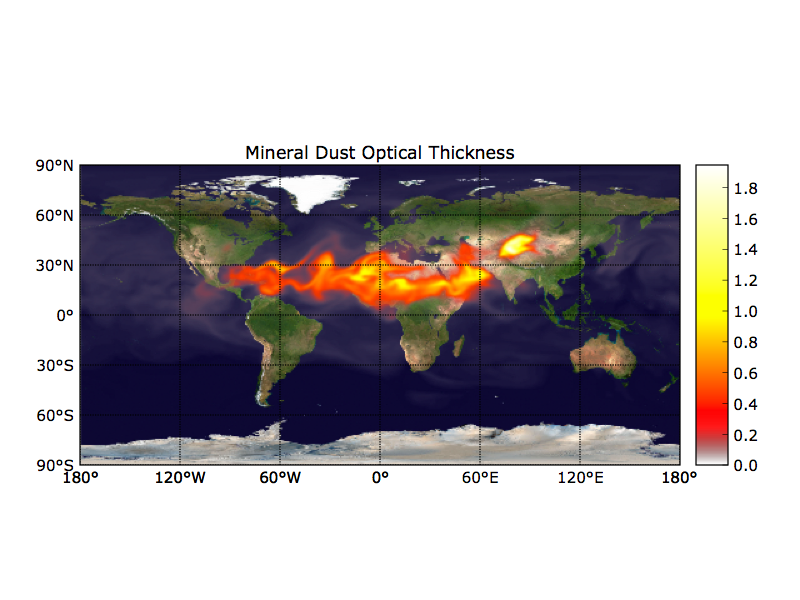# Bug or feature: bbox of maps

Hi,

(A similar issue was reported back in 7/4/11 without a definite solution, so I am reposting with some additional diagnostics. ) Up to Matplotlib 0.99 (EPD 6.3) the code snippet below produced a plot with an aligned colorbar as in the attached “correct.png” plot:

``````    fig = plt.figure()
m = Basemap(..., ax=ax, ...)
im = m.imshow(...)

``````
``````# show()
``````
``````    # setup colorbar axes and draw colorbar
bbox = ax.get_position()
l,b,w,h = bbox.bounds
cax = fig.add_axes([l+w+0.05, b, 0.05, h],frameon=False)
fig.colorbar(im, cax=cax)
``````

Starting with Matplotlib 1.0.0 this no longer works. The ax.get_position() no longer returns the bounding box of the plot but the bbox of the window, see the attached “wrong.png” attached. Some odd behavior:

1. I can get the code above to work correctly under MPL 1.0 if I uncomment the show() line above. (This is not an acceptable solution because show() blocks if not in interactive mode).

2. Under ipython, if after plotting I print gca().get_position().bounds I get the correct bounding box, even when I just got the wrong colorbar positioning.

3. If I run the code above twice in a row (without creating a new fig), the second time around the correct bounding box is returned.

Can someone explain to me what is going on? Is this one of those arcane features of matplotlib transform caching? It appears that show() is flushing some type of buffer. Is there any way of accomplishing this without actually calling show()?

Thank you,

Arlindo···

Arlindo da Silva
dasilva@…3027…